## Section38.8Force on Current Carrying Wire

Consider placing a straight metallic wire of length $L$ carrying current $I$ in a magnetic field $\vec B\text{.}$ Below, we will present arguments that will show that net force on an electric current in a straight wire is given by the following cross product of two vectors.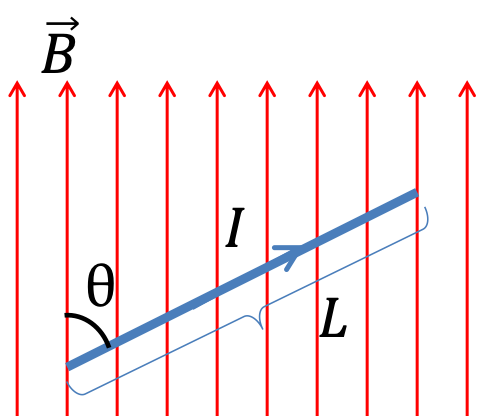\begin{equation} \vec F_m = I\vec L \times \vec B,\label{eq-force-on-current-carrying-wire-result}\tag{38.8.1} \end{equation}

where displacement vector $\vec L$ has length $L$ with direction in the direction of current flow. We can write the magnitude and direction of the force as follows.

\begin{equation*} \vec F_m = \begin{cases} I L B \sin\,\theta \amp (\text{magnitude})\\ \text{right-hand rule} \amp (\text{direction}) \end{cases} \end{equation*}

We have already seen the right-hand rule that gives the direction of $\vec F_m$ in Figure 38.4.3 and Figure 38.4.4, where $v$ is in the direction of current $I\text{.}$ In Figure 38.8.1, the right-hand rule for current in a metallic wire is illustrted.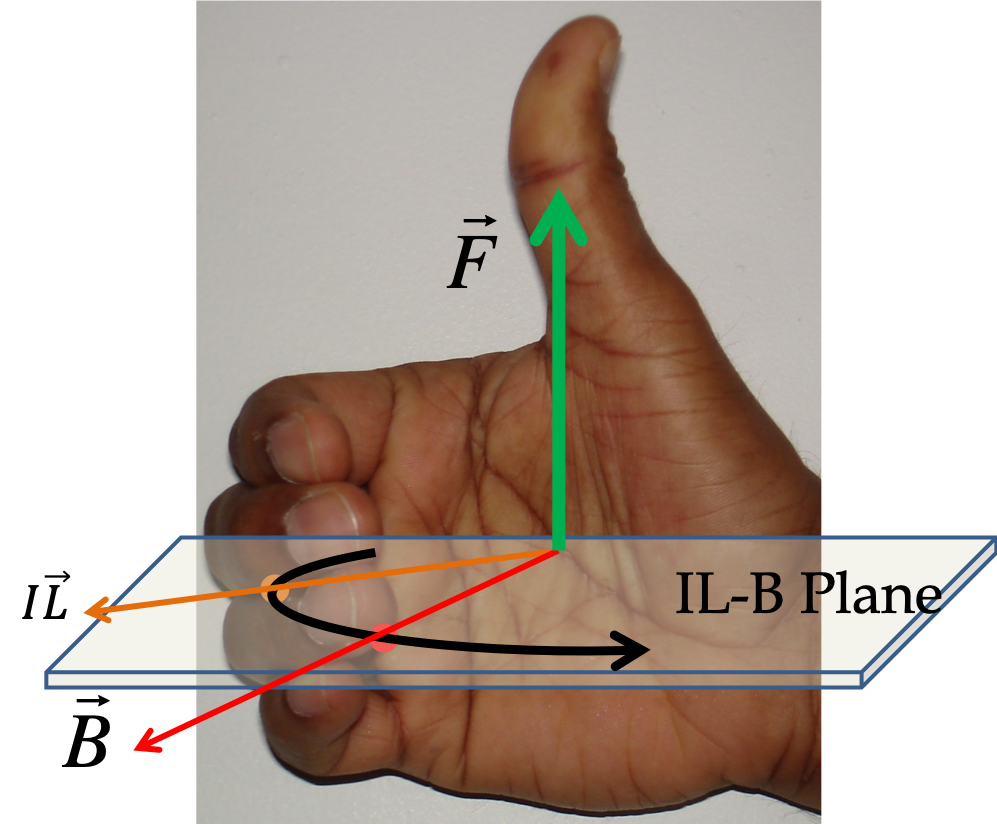Figure 38.8.1. Right-hand rule for magnetic force on a wire carrying current. Two ways to use the Right-hand Rule. Left panel: Place the wire displacement in the current direction and magnetic field in one plane, and then, sweep from current towards magnetic field with right hand; magnetic force is directed towards the thumb and perpendicular to the plane of current and magnetic field; Right panel: Orient your right palm such that current is in the direction of the thumb and magnetic field towards any other finger; magnetic force will be coming out and perpendicular to the palm.

### Subsection38.8.1Derivation of Force on Current in Straight Wire

Let us see how we can obtain Eq. (38.8.1) from conduction electron model of a metallic. Let $n$ be the number of conduction electrons per unit volume, $A$ the cross-sectional area, and $\vec v$their average drift velocity. The total charge of electrons in length $L$ of the wire that are moving is

\begin{equation*} Q = -e n A L. \end{equation*}

Since they are moving with average velocity $\vec v\text{,}$ the force on them is

\begin{equation} \vec F_m = Q\vec v \times B = -enAL \vec v \times \vec B.\label{eq-magnetic-force-on-electrons-in-current-1}\tag{38.8.2} \end{equation}

In terms of the current carrier density, current $I$ will be

\begin{equation*} I = enAv. \end{equation*}

Now, we define a displacement vector $\vec L$ to point in the direction opposite to the direction of electron's velocity, but with magnitude to be the length of the wire. Then, using this vector, we can also write factors in Eq. (38.8.2) as follows.

\begin{equation*} -enAL \vec v = enAv\vec L = I \vec L. \end{equation*}

Therefore, we will write the magnetic force on electric current in a straight wire to be

\begin{equation} \vec F_m = I \vec L \times \vec B.\label{eq-magnetic-force-on-electrons-in-current-2}\tag{38.8.3} \end{equation}

### Subsection38.8.2Effective Force on the Wire vs Magnetic Force on the Current

Suppose you place a metal wire at rest in a magnetic field. Then, when you turn current on through the wire, the wire gains kinetic energy. How's that possible, if force on a current-carrying wire is from magnetic force on moving electrons and magnetic force on moving charges cannot change speed since force is perpendicular to the direction of velocity?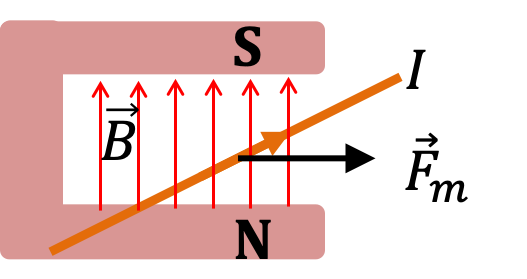You may recall that no work is done on moving isolated charge by magnet. Then, it appears odd that a magnet can make a current carrying wire move, especially, if we want to interpret force on current in terms of force on individual conduction electrons. The resolution of this paradox comes from looking at force on atoms of wire, not just on conduction electrons.

Say, conduction electrons are moving along the wire, then, magnetic force on them will impart an acceleration to these electrons in the direction perpendicular to the wire and deflect them towards the side of the wire. But, there is no where futher to go in the material. That would mean the atoms of the material gain a side-way momentum component.

The force responsible for collision between conduction electrons and atoms are electrical in nature and can do work. Therefore, although, magnetic forces on the conduction electrons do not do any work, the inertial force on the atoms can do work.

Even though, we refer the force on the wire as “magnetic force”, we should be aware that this use of the term magnetic force leads to inconsistencies with the basic observation that magnetic forces cannot do work on moving charges. The main point here is that although the force on conduction electrons is magnetic, the net force on the wire in a magnetic field is not magnetic.

### Subsection38.8.3Force on a Wire with Multiple Straight Segments

Magnetic force on a wire that bends around we just have to compute force on each straight segment and add them vectorially. Figure 38.8.2 shows an $\text{L-shaped}$ part of a circuit that is in a magnetic field, with lengths of segments $a$ and $b\text{.}$ The magnitude of forces on the two segments are

\begin{align*} F_\text{on a} \amp = I a B,\\ F_\text{on b} \amp = I b B, \end{align*}

Force on the bottom segment, $\vec F_\text{on a}\text{,}$ is pointed up as you can check by using the Right-Hand rule and force on the left segment, $\vec F_\text{on b}\text{,}$ is pointed to the right. We need to add these force vectorially. Let us $x$ and $y$ axes as shown in the figure.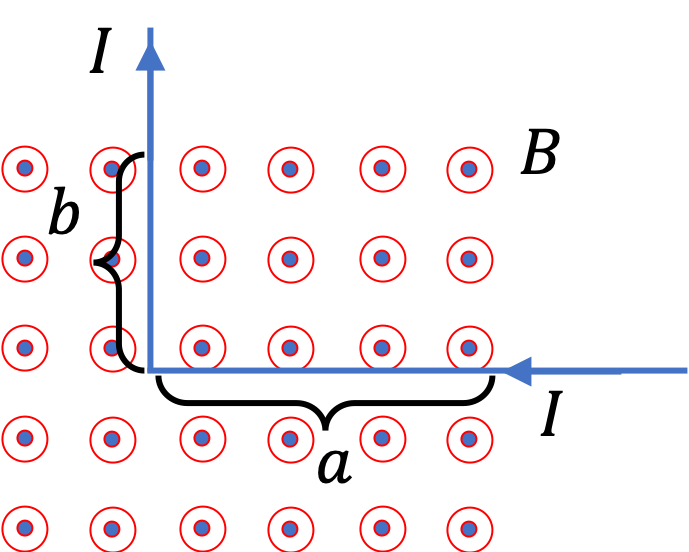Figure 38.8.2. Left panel: L-shaped part of a circuit with current $I$ in a magnetic field $\vec B\text{,}$ which is pointed out of page. Right panel: Forces on the two segments are in different diretions. The net magnetic force on the wires will be a vector sum, $\vec F_\text{on a} + \vec F_\text{on b}\text{,}$ of these two forces.

Adding the forces gives the following for the $x$ and $y$ components of the net force.

\begin{align*} F_x \amp = F_\text{on b} = IbB\\ F_y \amp = F_\text{on a} = IaB \end{align*}

The magnitude of the net force will be

\begin{equation*} F = \sqrt{F_x^2 + F_y^2} = IB\sqrt{a^2 + b^2}, \end{equation*}

and the direction as counterclockwise angle, $\theta$ from the postive $x$ axis will be

\begin{equation*} \theta = \tan^{-1}\left( \dfrac{F_y}{F_x} \right) = \tan^{-1}\left( \dfrac{a}{b} \right). \end{equation*}

### Subsection38.8.4(Calculus) Magnetic Force on Arbitrary Wire

Consider a curved amooth wire of arbitray shape carrying current $I$ in a magnetic field $\vec B$ as shown in Figure 38.8.3, which may also vary in space. You may already know that at the infintesimal level, a smooth curve looks straight. Therefore, we apply the force law on straight infintesimal segments and add them to get the general formula.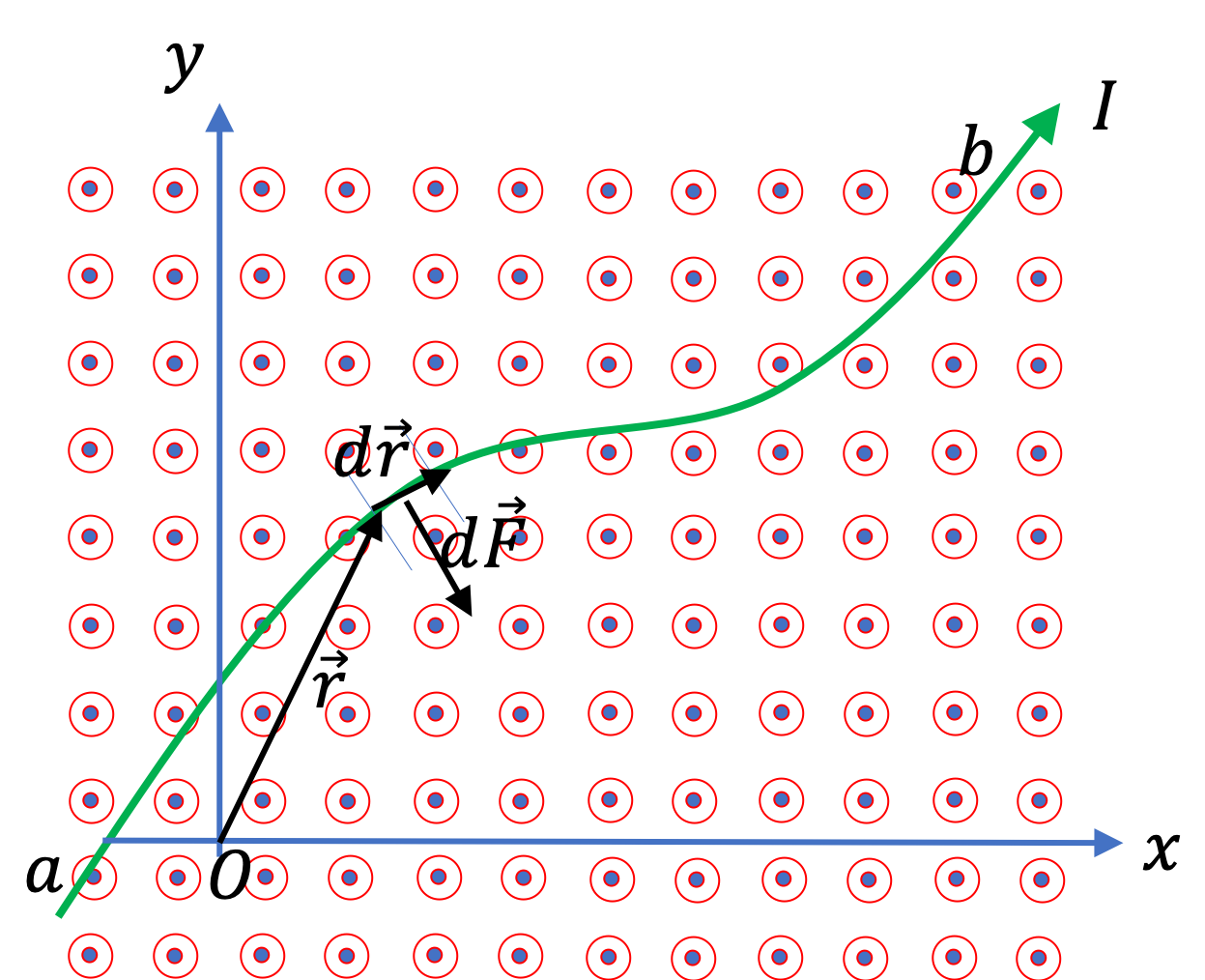Figure 38.8.3. Force on an element $d\vec r$ of an arbitrary shape wire, $d\vec F_m = I\, d\vec r\times \vec B\text{.}$

Let $\vec r$ be the location of an elment of the wire $d\vec r\text{,}$ whose length is $dr$ and direction in the tangent to the curve at that location. Let us denote the magnetic field at this location as $\vec B(\vec r)\text{.}$ Therefore, we have the following for the force on this infinitesimal piece of the wire.

\begin{equation*} d\vec F_m = I\, d\vec r \times \vec B(\vec r). \end{equation*}

Summing over infinitesimal segment is the integral from one end of the wire, point $a$ to the other end at point $b\text{.}$

\begin{equation*} \vec F_m = \int_{a}^{b}I\, d\vec r \times \vec B(\vec r). \end{equation*}

When applying this formula to a problem, you will have to set up the problem and “discover” the integral you need to perform, by making sure that $d\vec r$ is a vector along the wire. If wire has straight segments, Cartesian coordinates are helpful, and when wire is circular, polar coordinates are useful.

A $9\text{-cm}$ wire carrying a current of $5\text{ A}$ bends at $90^{\circ}\text{,}$ with one leg being $6\text{-cm}$ long and the other leg $3\text{-cm}$ long. The wire is in a region of uniform magnetic field of $2.5\text{ T}$ perpendicular to the plane of the wire. Find magnetic force on the wire.

Hint

$0.84\text{ N}\text{,}$ directed $63.5^{\circ}$ below the positive $x$ axis in the 4th quadrant where the $x$ axis is along $6\text{-cm}$ leg and the $y$ axis along $3\text{-cm}$ leg.

Solution

The magnetic forces on the two parts of the wire are shown in the figure. Their magnitudes as well as directions are different. We shall use the coordinate directions shown for adding the vectors analytically. The magnitudes of the forces are:

\begin{align*} F_{1} \amp = 5\:\text{A}\times 0.06\:\text{m}\times 2.5\:\text{T} = 0.75\text{ N}, \\ F_{2} \amp = 5\:\text{A}\times 0.03\:\text{m}\times 1.5\:\text{T} = 0.375\text{ N}, \end{align*}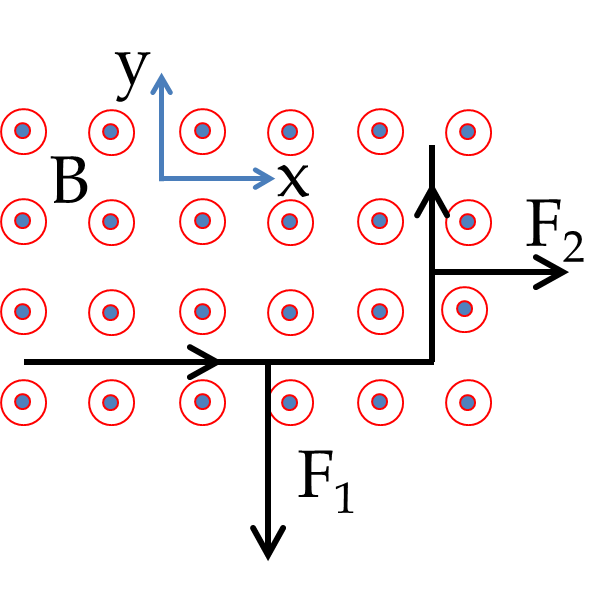The net force is not $(0.75\text{ N}+0.375\text{ N})$ since the forces are not in the same direction. We find that $x$ and $y$ components of the forces are

\begin{align*} \amp F_{1x} = 0,\ \ F_{1y} = - 0.75\:\text{N},\\ \amp F_{2x} = 0.375\:\text{N},\ \ F_{2y} = 0. \end{align*}

Therefore, $x$ and $y$ components of the net force are

\begin{equation*} F_x = 0.375\:\text{N},\ \ F_y = - 0.75\:\text{N}. \end{equation*}

The magnetic force has a magnitude of $\sqrt{0.75^2 + 0.375^2} = 0.84\:\text{N}$ and is pointed in the 4-th quadrant at an angle of $63.4^{\circ}$ clockwise from the positive $x$ axis.

A $10\text{-cm}$ wire carrying a current of $5\text{ A}$ bends at $30^{\circ}\text{,}$ with one leg being $6\text{-cm}$ long and the other leg $4\text{-cm}$ long. The wire is in a region of uniform magnetic field of $2.0\text{ T}$ perpendicular to the plane of the wire. Find magnetic force on the wire.

Hint

$0.32\:\text{N}$ at $51.3^{\circ}$ clockwise from the positive $x$ axis.

Solution

By using the formula $\vec F_m = I\vec L\times \vec B$ on each straight segment, we find that the magnitudes of the forces on them are

\begin{align*} F_{1} \amp = 5\:\text{A}\times 0.06\:\text{m}\times 2.0\:\text{T} = 0.6\text{ N}.\\ F_{2} \amp = 5\:\text{A}\times 0.04\:\text{m}\times 2.0\:\text{T}=0.4\text{ N}. \end{align*}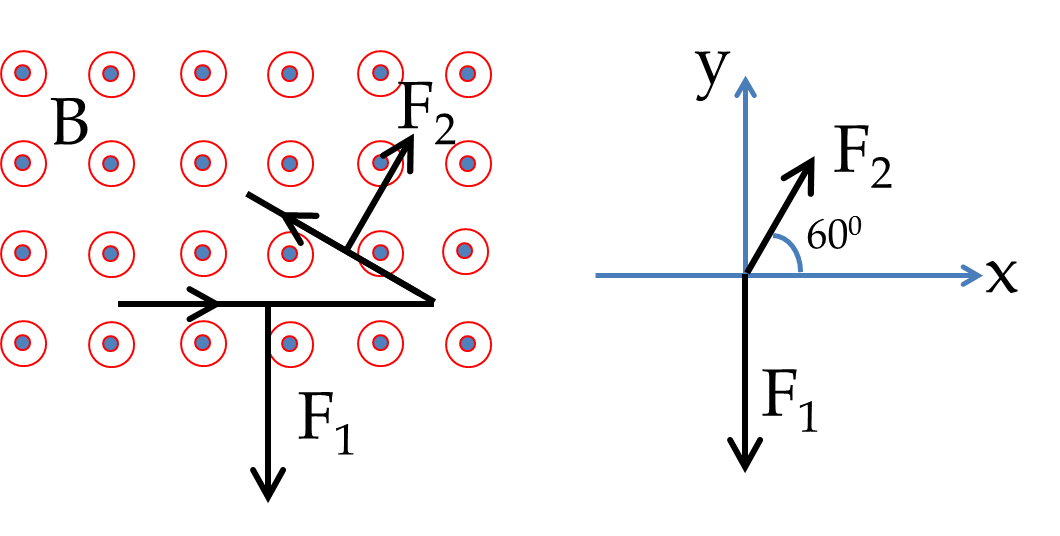The net force is not $(0.6\text{ N} + 0.4\text{ N})$ since the forces are not in the same direction. We need to add them vectorially. We will do that analytically by using the coordinate system shown in the figure. The $x$ and $y$ components of the forces $\vec F_1$ and $\vec F_2$ are

\begin{align*} \amp F_{1x} = 0,\ \ F_{1y} = - 0.6\:\text{N}. \\ \amp F_{2x} = 0.2 \:\text{N},\ \ F_{2y} = 0.35\:\text{N}, \end{align*}

The $x$ and $y$ components of the net force are

\begin{equation*} F_x = 0.20\:\text{N},\ \ F_{y} = -0.25\:\text{N}. \end{equation*}

The magnetic force has a magnitude of

\begin{equation*} \sqrt{0.20^2 + 0.25^2} = 0.32\:\text{N}, \end{equation*}

and is pointed in the 4-th quadrant at an angle of $51.3^{\circ}$ clockwise from the positive $x$ axis.

Current flows in a loop shaped as a square of side $a\text{.}$ The loop of wire is in a plane perpendicular to a magnetic field of magnitude $B$ as shown in the figure. What is the magnetic force on the full loop?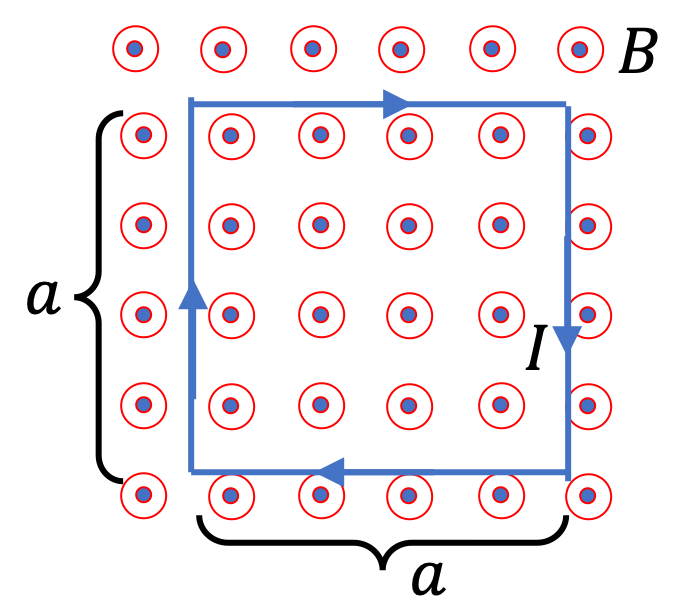Hint

Look at the direction of forces on the four straight segments.

$0\text{.}$

Solution

Based on the given orientation of current and magnetic field, the forces on the four straight segments have equal magnitude, $F_i = I a B\text{,}$ $i = 1,\ 2\, 3\, 4\text{.}$ But their directions are such that $\vec F_1$ cancels $\vec F_3$ and $\vec F_2$ cancels $\vec F_4\text{.}$ Therefore, net force on the loop is zero.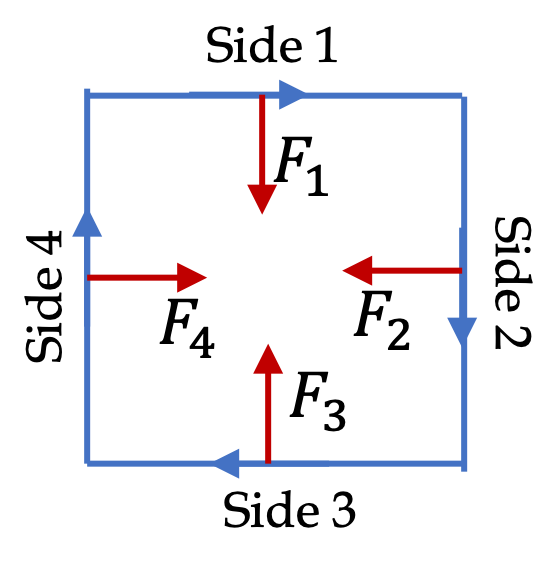Current flows in a loop shaped as a square of side $a\text{.}$ The loop of wire is in a plane that is not perpendicular to a magnetic field of magnitude $B$ but slanted as shown in the figure. What is the magnetic force on the full loop?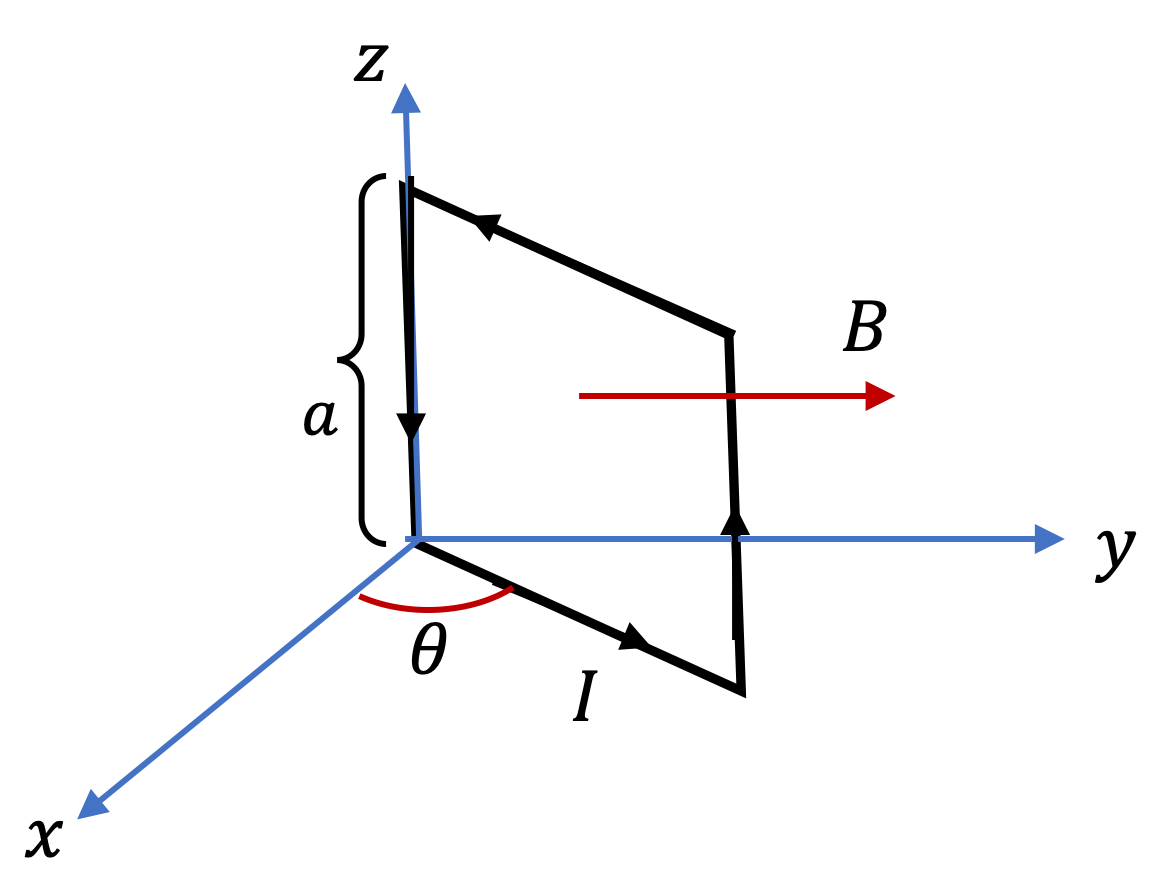Hint

Find pairs of forces that cancel. Its easier to work with cross product.

$0\text{.}$

Solution

Based on the given orientation of current and magnetic field, the forces on various segments have the following magnitudes and directions, which give the net force to be zero. Here I write directions using the unit vectors, $\hat i, \hat j, \hat k\text{,}$ along the Cartesian axes.

\begin{align*} \amp \vec F_3 = I a B\hat k = - \vec F_1 \\ \amp \vec F_4 = I a B \cos\,\theta\hat i = - \vec F_2 \end{align*}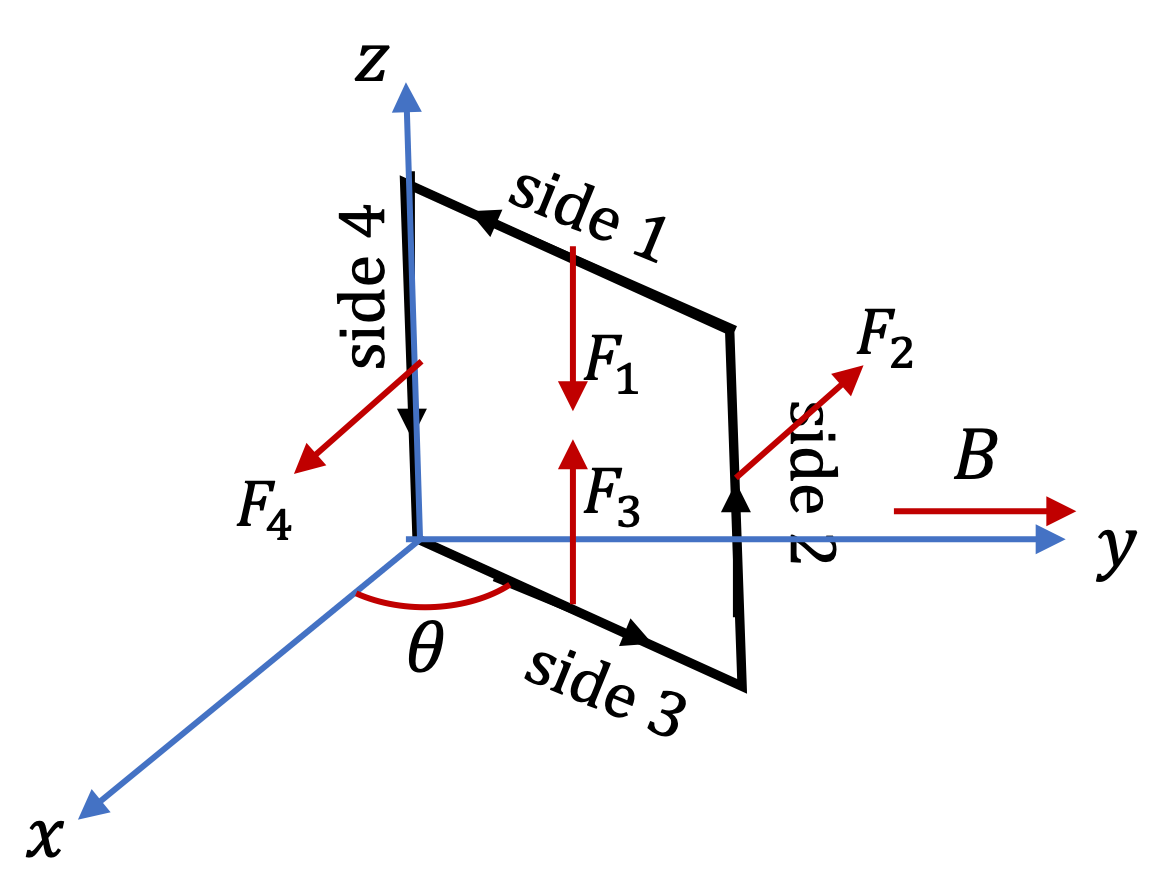I got these from using the following by using $\vec B = B\hat j$ and the following vectors that go into $I\vec L\times \vec B$ for force on each segment.

\begin{align*} \vec L_3 \amp = a\,\cos\theta\, \hat i + a\,\sin\theta\,\hat j = -\vec L_1\\ \vec L_2 \amp = a \hat k = -\vec L_4 \end{align*}

A straight wire of length $15\text{ cm}$ is carrying a current of $20\text{ A}\text{.}$ The wire is placed on a table where there is a magnetic field whose magnitude varies over the length of the wire, but the direction is always perpendicular to the table facing up. If wire is along the $x$ axis and the direction of the magnetic field is the $y$ axis, the $y$-component of the magnetic field is given by the following function of the coordinate $x\text{.}$

\begin{equation*} B_y(x) = \frac{0.16}{x}\ \ \text{(T with } x \text{ in m)}. \end{equation*}

The wire is placed from $x = 1\text{ cm}$ to $x = 16\text{ cm}\text{.}$ Find the magnetic force on the wire.

Hint

Set up the integral for computing the force by looking at an infintesimal segment of the wire.

$8.9\:\text{N}$ and direction towards the positive $z$ axis.

Solution

Figure below shows a segment of length $\Delta x$ of a wire along $x$ axis. We will write down an expression for force on this segment and then try to add over all such segments.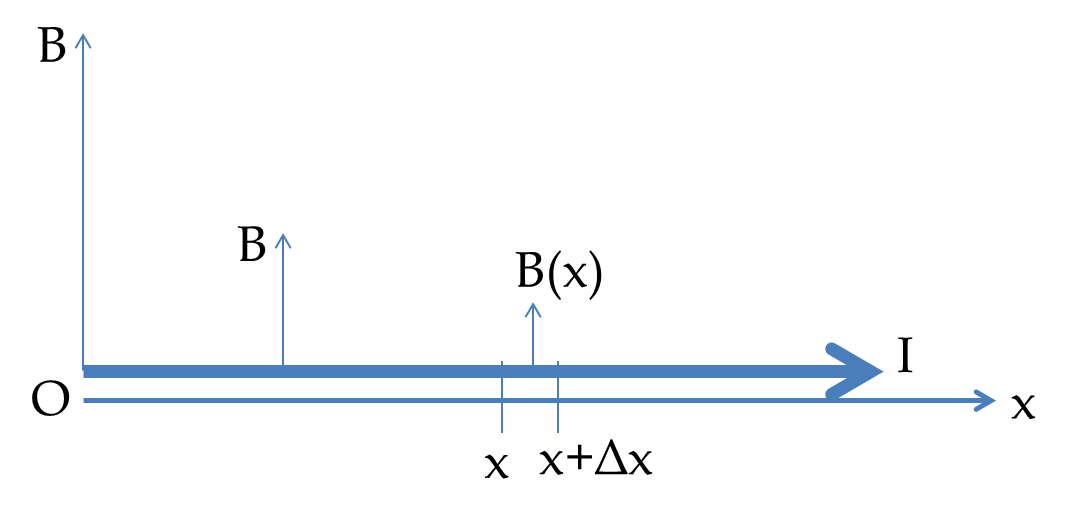The force on this segment between $x$ and $\Delta x$ is towards $z$ axis. Therefore, the $z$ component of the force will be

\begin{equation*} \Delta F_z = I\:\dfrac{0.16}{x}\:\Delta x. \end{equation*}

The total force will be obtained by adding contributions of force on each infinitesimal segment. This leads to an integration.

\begin{align*} F_z \amp = \int_{0.01}^{0.16}\: I \dfrac{0.16}{x}\: dx,\\ \amp = 20\:\text{A}\times 0.16\:\text{T.m}\times \ln(0.16/0.01) = 8.9\:\text{N}. \end{align*}

Since other components of force are zero, we find that the force on the wire has magnitude $8.9\:\text{N}$ and direction towards the positive $z$ axis.

A wire is bent into a quarter circle of radius $2\text{ cm}$ and placed between the poles of a magnet where there is a uniform magnetic field of $3.5\text{ T}$ perpendicular to the plane of the wire. Find force on the wire when there is $30\text{ A}$ current through it.

Hint

Use polar coordinates and look at force on an arc of length $Rd\theta\text{.}$

$2.95\text{ N}$ at $45^{\circ}$ from one end.

Solution

We will look at the infinitesimal element of the wire between angle $\theta$ and $\theta + d\theta\text{.}$ Length of this element is $Rd\theta\text{.}$ Theerefore, force on this element has the magnitude $dF = I B R d\theta$ and direction radially out as shown in the figure. Since the radial direction changes with $\theta$ we will write the force in terms of the $x$ and $y$ components.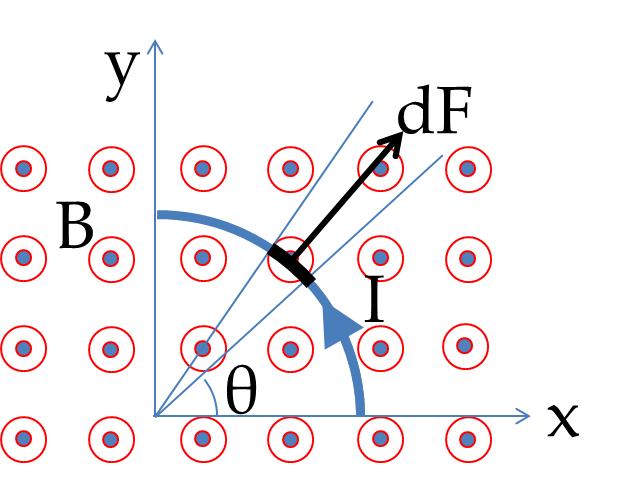\begin{equation*} dF_x = I B R \:\cos\theta\:d\theta,\ \ dF_y = I B R \:\sin\theta\:d\theta. \end{equation*}

Integrating these components from $\theta=0$ to $\theta = \dfrac{\pi}{2}$ gives the components of the net force.

\begin{equation*} F_x = \int_0^{\frac{\pi}{2}}\:I B R \:\cos\theta\:d\theta = IBR,\ \ F_y = IBR. \end{equation*}

Therefore, the force on the wire has the magnitude $\sqrt{2}\:IBR = 2.97\:\text{N}$ and direction $45^{\circ}$ from the positive $x$-axis.